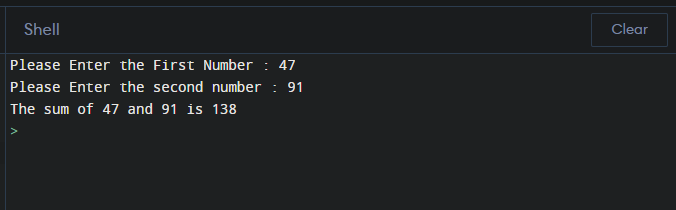Python Program to add Two numbers

How can we add two numbers in Python? We can add numbers using arithmetic operator "+" and print their sum using 'print' function.

Addition of two numbers in Python :

num1 = input("Please Enter the First Number : ")
num2 = input("Please Enter the second number : ")
sum = int(num1) + int(num2)
print('The sum of {0} and {1} is {2}'.format(num1, num2, sum))

Output :